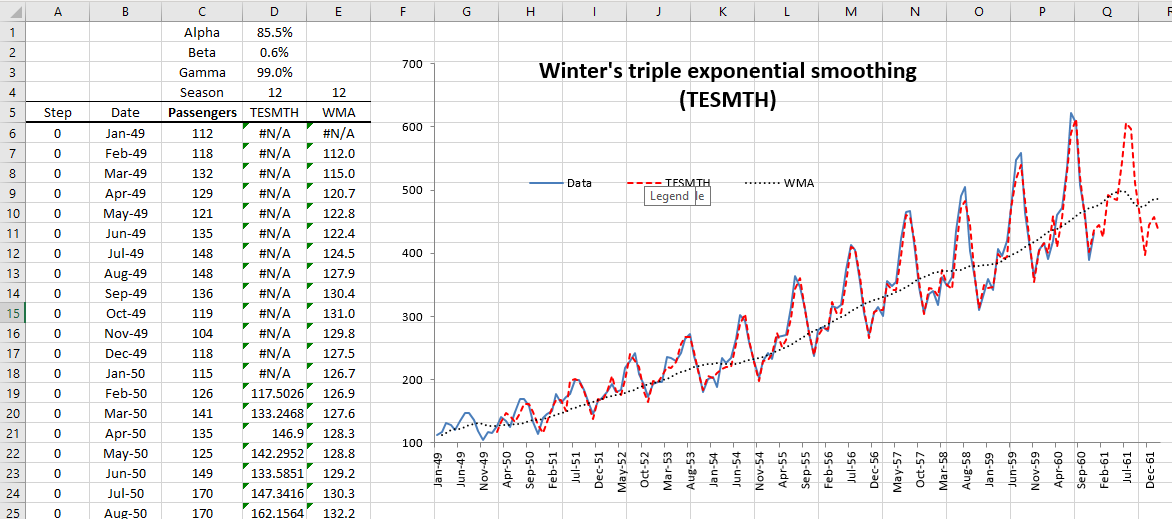# Holt-Winters' Triple Exponential Smoothing in Excel (TESMTH)

Check out our Holt-Winters' Triple Exponential Smoothing tutorial below. The playlist contains tutorials that teach you how to utilize the optimization switch and calculate with seasonal decomposition.

Optimization On Optimization Off Decomposition

## Optimization On

In this video, we show you how to use Holt's triple exponential smoothing function in NumXL with an optimization switch for smoothing factors.

Video script

Scene 1:

Hello and welcome to the exponential smoothing tutorial series. In this tutorial we'll resume the demonstration of Holt Winters Triple Exponential Smoothing functions and the built-in optimizer in NumXL. For sample data we are using the same monthly international passengers data set.

Let's begin by selecting the cell D11. Examine the cell formula in the formula toolbar. Notice we already have a call for the triple exponential smoothing function, so press F2 to edit, and then click on the FX button found on the left side of the equation toolbar.

Scene 2:

The triple exponential smoothing function argument's dialog box will pop up, and notice that the cell D4 is used for the optimize argument, so we don't need to change the formula just the cell D4. Now click cancel to go back to your worksheet.

Scene 3:

Select the cell D4 then type in true or one and hit enter when done.

Scene 4:

Since we have the automatic calculation on, all of the values of the smooth time series are recalculated using the optimal smoothing factors known as alpha beta and gamma. Notice the favorable change is in mean squared error, mean absolute scaled error, and symmetric mean absolute percentage error. The calibrated triple exponential smoothing function has a 22% lower mean absolute error than that of a naive reference model.

Now what about the alpha value in D1, the beta value in D2, and the gamma in D3? When the optimizer flag is turned on the function uses the value of alpha, beta, and gamma as a starting value for the optimizer. The NumXL optimizer is very robust such that you may leave the alpha beta and/or gamma blank and the optimizer will most likely return the same values.

Scene 5:

The triple exponential smoothing function calculates the optimal values for alpha and beta using the available information or data. The available data increases the time, so the function calculates a new value for each step. Let's examine the values of those parameters, so select the cell E11. Start typing the triple exponential smoothing function =TESMTH(. Once the function is found click on the FX button found on the left side of the equation toolbar.

Scene 6:

This will invoke the function arguments dialog box for the triple exponential smoothing function. Select the input cell range, this is the same cell range we used earlier for the forecasting column D and then lock the cell reference by pressing F4. Enter a value of true or one in the order field.

For the initial value of alpha let's use a value in D1 and then lock the cell by pressing F4. For the initial value for beta let's use the value in D2 and then lock the cell by pressing F4. For the initial value for gamma let's use the value in D3 and then lock the cell by pressing F4. For the season length or duration let's use a value in D5 and then lock the cell by pressing F4. For the optimize switch let's use the value in cell D3 and then lock the cell by pressing F4. Set the forecast time to zero or set it to the value in A11 then lock the cell for column movement. For the return type, type in one for returning the value of the level smoothing parameter alpha then click OK.

Scene 7:

The built-in optimizer requires a few non missing observations to run or it will return the starting alpha value as it is the case here. Copy the formulas to the cells below it then plot the optimal value for the level smoothing parameter, alpha.

Scene 8:

Let's repeat the same procedure for beta so select the cell in F11 and start typing =TESMTH( and when the function is found click on the FX button found on the left side of the equation toolbar.

Scene 9:

Use the same input like earlier for all of the arguments except for return type. For the return type, type in two for returning the value of the transmitting parameter beta and then click OK.

Scene 10:

Similar to alpha the built-in optimizer requires a few non missing observations to run or it will return the same starting beta value as it is the case here. Copy the formula to the cells below it and then plot the optimal beta values throughout the sample. Let's repeat the same procedure for gamma, so select the cell in G11 and start typing =TESMTH( and when the function is found click on the FX button found on the left side of the equation toolbar.

Scene 11:

Use the same input like earlier for all arguments except for return type. For the return type, type in 3 for returning the value of the seasonal indices smoothing parameter, gamma, and then click OK.

Scene 12:

Similar to the case of alpha and beta, the built-in optimizer requires a few non-missing observations to run or it will return the same starting gamma value as is the case here. Copy the formula to the cells below it and then plot the optimal gamma values throughout the sample.Scene 13:

That's all for now, thank you for watching!